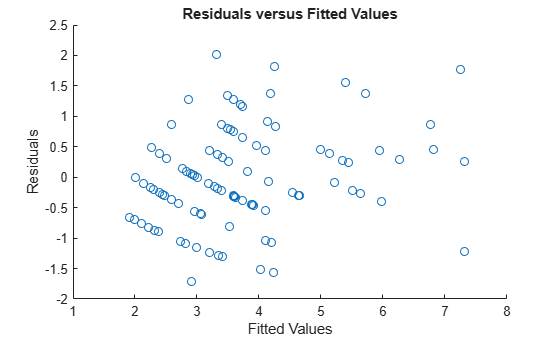Main Content

# residuals

Residuals of fitted generalized linear mixed-effects model

## Syntax

``r = residuals(glme)``
``r = residuals(glme,Name,Value)``

## Description

````r = residuals(glme)` returns the raw conditional residuals from a fitted generalized linear mixed-effects model `glme`.```

example

````r = residuals(glme,Name,Value)` returns the residuals using additional options specified by one or more `Name,Value` pair arguments. For example, you can specify to return Pearson residuals for the model.```

## Input Arguments

expand all

Generalized linear mixed-effects model, specified as a `GeneralizedLinearMixedModel` object. For properties and methods of this object, see `GeneralizedLinearMixedModel`.

### Name-Value Pair Arguments

Specify optional comma-separated pairs of `Name,Value` arguments. `Name` is the argument name and `Value` is the corresponding value. `Name` must appear inside quotes. You can specify several name and value pair arguments in any order as `Name1,Value1,...,NameN,ValueN`.

Indicator for conditional residuals, specified as the comma-separated pair consisting of `'Conditional'` and one of the following.

ValueDescription
`true`Contributions from both fixed effects and random effects (conditional)
`false`Contribution from only fixed effects (marginal)

Conditional residuals include contributions from both fixed- and random-effects predictors. Marginal residuals include contribution from only fixed effects. To obtain marginal residual values, `residuals` computes the conditional mean of the response with the empirical Bayes predictor vector of random effects, b, set to 0.

Example: `'Conditional',false`

Residual type, specified as the comma-separated pair consisting of `'ResidualType'` and one of the following.

Residual TypeConditionalMarginal
`'raw'`

`${r}_{ci}={y}_{i}-{g}^{-1}\left({x}_{i}^{T}\stackrel{^}{\beta }+{z}_{i}^{T}\stackrel{^}{b}+{\delta }_{i}\right)$`

${r}_{mi}={y}_{i}-{g}^{-1}\left({x}_{i}^{T}\stackrel{^}{\beta }+{\delta }_{i}\right)$

`'Pearson'`

`${r}_{ci}^{pearson}=\frac{{r}_{ci}}{\sqrt{\frac{\stackrel{^}{{\sigma }^{2}}}{{w}_{i}}{v}_{i}\left({\mu }_{i}\left(\stackrel{^}{\beta },\stackrel{^}{b}\right)\right)}}$`

${r}_{mi}^{pearson}=\frac{{r}_{mi}}{\sqrt{\frac{\stackrel{^}{{\sigma }^{2}}}{{w}_{i}}{v}_{i}\left({\mu }_{i}\left(\stackrel{^}{\beta },0\right)\right)}}$

In each of these equations:

• yi is the ith element of the n-by-1 response vector, y, where i = 1, ..., n.

• g-1 is the inverse link function for the model.

• xiT is the ith row of the fixed-effects design matrix X.

• ziT is the ith row of the random-effects design matrix Z.

• δi is the ith offset value.

• σ2 is the dispersion parameter.

• wi is the ith observation weight.

• vi is the variance term for the ith observation.

• μi is the mean of the response for the ith observation.

• $\stackrel{^}{\beta }$ and $\stackrel{^}{b}$ are estimated values of β and b.

Raw residuals from a generalized linear mixed-effects model have nonconstant variance. Pearson residuals are expected to have an approximately constant variance, and are generally used for analysis.

Example: `'ResidualType','Pearson'`

## Output Arguments

expand all

Residuals of the fitted generalized linear mixed-effects model `glme` returned as an n-by-1 vector, where n is the number of observations.

## Examples

expand all

Load the sample data.

`load mfr`

This simulated data is from a manufacturing company that operates 50 factories across the world, with each factory running a batch process to create a finished product. The company wants to decrease the number of defects in each batch, so it developed a new manufacturing process. To test the effectiveness of the new process, the company selected 20 of its factories at random to participate in an experiment: Ten factories implemented the new process, while the other ten continued to run the old process. In each of the 20 factories, the company ran five batches (for a total of 100 batches) and recorded the following data:

• Flag to indicate whether the batch used the new process (`newprocess`)

• Processing time for each batch, in hours (`time`)

• Temperature of the batch, in degrees Celsius (`temp`)

• Categorical variable indicating the supplier (`A`, `B`, or `C`) of the chemical used in the batch (`supplier`)

• Number of defects in the batch (`defects`)

The data also includes `time_dev` and `temp_dev`, which represent the absolute deviation of time and temperature, respectively, from the process standard of 3 hours at 20 degrees Celsius.

Fit a generalized linear mixed-effects model using `newprocess`, `time_dev`, `temp_dev`, and `supplier` as fixed-effects predictors. Include a random-effects term for intercept grouped by `factory`, to account for quality differences that might exist due to factory-specific variations. The response variable `defects` has a Poisson distribution, and the appropriate link function for this model is log. Use the Laplace fit method to estimate the coefficients. Specify the dummy variable encoding as `'effects'`, so the dummy variable coefficients sum to 0.

The number of defects can be modeled using a Poisson distribution

`${\text{defects}}_{ij}\sim \text{Poisson}\left({\mu }_{ij}\right)$`

This corresponds to the generalized linear mixed-effects model

`$\mathrm{log}\left({\mu }_{ij}\right)={\beta }_{0}+{\beta }_{1}{\text{newprocess}}_{ij}+{\beta }_{2}{\text{time}\text{_}\text{dev}}_{ij}+{\beta }_{3}{\text{temp}\text{_}\text{dev}}_{ij}+{\beta }_{4}{\text{supplier}\text{_}\text{C}}_{ij}+{\beta }_{5}{\text{supplier}\text{_}\text{B}}_{ij}+{b}_{i},$`

where

• ${\text{defects}}_{ij}$ is the number of defects observed in the batch produced by factory $i$ during batch $j$.

• ${\mu }_{ij}$ is the mean number of defects corresponding to factory $i$ (where $i=1,2,...,20$) during batch $j$ (where $j=1,2,...,5$).

• ${\text{newprocess}}_{ij}$, ${\text{time}\text{_}\text{dev}}_{ij}$, and ${\text{temp}\text{_}\text{dev}}_{ij}$ are the measurements for each variable that correspond to factory $i$ during batch $j$. For example, ${\text{newprocess}}_{ij}$ indicates whether the batch produced by factory $i$ during batch $j$ used the new process.

• ${\text{supplier}\text{_}\text{C}}_{ij}$ and ${\text{supplier}\text{_}\text{B}}_{ij}$ are dummy variables that use effects (sum-to-zero) coding to indicate whether company `C` or `B`, respectively, supplied the process chemicals for the batch produced by factory $i$ during batch $j$.

• ${b}_{i}\sim N\left(0,{\sigma }_{b}^{2}\right)$ is a random-effects intercept for each factory $i$ that accounts for factory-specific variation in quality.

```glme = fitglme(mfr,'defects ~ 1 + newprocess + time_dev + temp_dev + supplier + (1|factory)',... 'Distribution','Poisson','Link','log','FitMethod','Laplace','DummyVarCoding','effects');```

Generate the conditional Pearson residuals and the conditional fitted values from the model.

```r = residuals(glme,'ResidualType','Pearson'); mufit = fitted(glme);```

Display the first ten rows of the Pearson residuals.

`r(1:10)`
```ans = 10×1 0.4530 0.4339 0.3833 -0.2653 0.2811 -0.0935 -0.2984 -0.2509 1.5547 -0.3027 ```

Plot the Pearson residuals versus the fitted values, to check for signs of nonconstant variance among the residuals (heteroscedasticity).

```figure scatter(mufit,r) title('Residuals versus Fitted Values') xlabel('Fitted Values') ylabel('Residuals')```The plot does not show a systematic dependence on the fitted values, so there are no signs of nonconstant variance among the residuals.

Download ebook